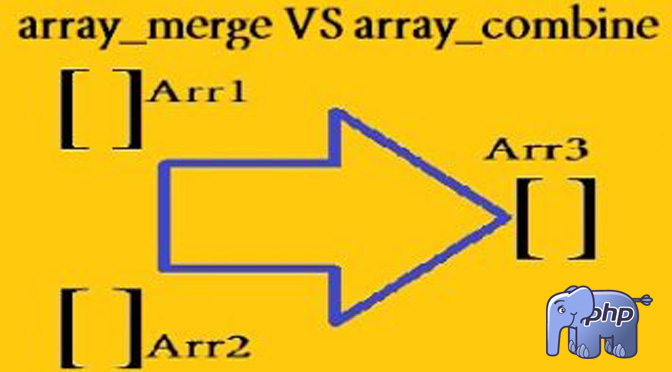# what is difference between array_merge and array_combine in phpDifference between array_merge and array_combine in php

Array Merge

PHP: array_merge
Merge one or more arrays

Merges the elements of one or more arrays together so that the values of one are appended to the end of the previous one.
If the input arrays have the same string keys, then the later value for that key will overwrite the previous one or if the arrays contain numeric keys, then the later value will not overwrite the original value and will be appended.

Values in the input array with numeric keys will be renumbered with incrementing keys starting from zero in the result array.

Have a look on example below

Example:
<?php
\$array1 = array(“color” => “red”, 2, 4);
\$array2 = array(“a”, “b”, “color” => “green”, “shape” => “trapezoid”, 4);
\$result = array_merge(\$array1, \$array2);
print_r(\$result);
?>

Output will:
Array
(
[color] => green
 => 2
 => 4
 => a
 => b
[shape] => trapezoid
 => 4
)

Array Combine

PHP: array_combine
Creates an array by using one array for keys and another for its values

Example:
<?php
\$array1 = array(“green”,”red”,”yellow”);
\$result = array_combine(\$array1, \$array2);
print_r(\$result);
?>

Output will:
Array
(
1.Jaydipsinh says: